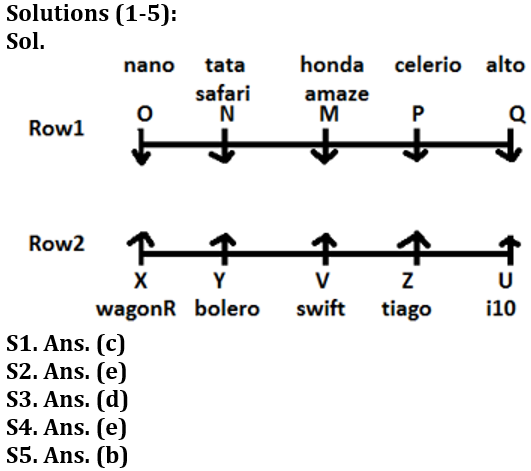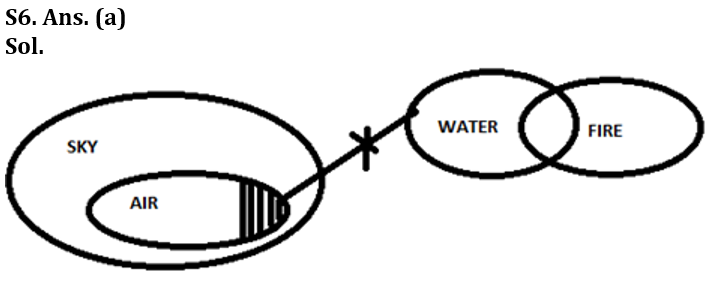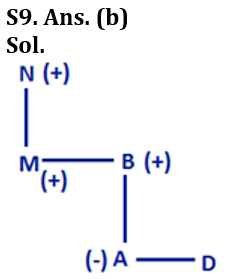Latest Banking jobs   »

# Reasoning Ability Quiz For LIC AAO Mains 2023-3rd March

Directions (1-5): Study the following information carefully and answer the questions:
Ten cars are i.e., M, N, O, P, Q, U, V, X, Y and Z parked in two parallel rows (not necessarily in same order). In 1st row M, N, O, P and Q cars are parked facing south. In 2nd row, U, V, X, Y and Z are parked facing north. Therefore, in the given seating arrangement, each car parked in a row faces another car of the other row. All cars are of different brands i.e., Honda Amaze, Bolero, Tata safari, Nano, Celerio, Alto, I10, Swift, Wagon R, and Tiago (not necessarily in same order).
Car M is not Nano and Car P is Celerio. Car Y is parked third to the left of Car U which is I10. Car M faces the car which is next of Car Y which is Bolero. The one which is Wagon R is parked at extreme end. Car O is parked second to the right of Car M. The one which is Honda Amaze faces the one which is Swift, but Car M is not swift. Only one car is parked between Car N and Car P. Car V and Car Z are parked next to each other. Car Z does not face Car M and Car N which is not Tiago. The one which faces Car U is alto. The one which faces car next to Car Y is Nano.

Q1. How many cars are parked between Car N and Alto?
(a) None
(b) One
(c) Two
(d) Three
(e) None of these

Q2. Which among the following faces Car P?
(a) U
(b) The one which is swift
(c) X
(d) N
(e) The one which is Tiago

Q3. Which of the following is true regarding Car M?
(a) Car N and Car X are next to Car M
(b) Car M is parked at one of the extreme ends.
(c) Car M is Celerio.
(d) Car P is parked immediate left of Car M
(e) None of these

Q4. Which among the following pair of cars is parked exactly in the middle of the rows?
(a) M, Z
(b) P, Y
(c) None of these
(d) U, N
(e) M, V

Q5. V is which of the following brand?
(a) Nano
(b) Swift
(c) Celerio
(d) Alto
(e) None of these

Directions (6): In the following question three statements are followed by some conclusions. You have to take the given statements to be true even if they seem to be at variance from commonly known facts. Read all the conclusions and then decide which of the given conclusions logically follows from the given statements disregarding commonly known facts.

Q6. Statements: All Air are Sky.
Some Air are not Water
Some Water are Fire
Conclusions: I. Some Sky are not Water
II. No Fire is Sky
III. Some water can be sky
(a) Only I and III follow
(b) Only I and II follow
(c) Only III and II follow
(d) None of these.
(e) Only I follow.

Q7. In the word ‘TRUNCATED’ all consonants are written as their preceding letter and all vowels are written as their following letters. Now all letters are arranged in alphabetical order from left to right and all the repeated letters are eliminated. Then, how many such pairs of letters are there, each of which have as many letters between them in the word (in both forward and backward direction) as they have between them in the English alphabetical series?
(a) One
(b) Two
(c) Four
(d) Three
(e) More than four

Q8. If we form the word by 1st and 3rd letter of ‘LOCKDOWN’ and 2nd, 4th and 6th letter of ‘UPDATES’ then, what will be the 3rd letter from right?
(a) E
(b) P
(c) L
(d) A
(e) None of these

Q9. If A + B means A is the mother of B; A – B means A is the brother B; A % B means A is the father of B and A x B means A is the sister of B, which of the following shows N is the paternal grandfather of A?
(a) N + M ─ B % A × D
(b) N % M ─ B % A × D
(c) N + M ─ B % D × A
(d) N % M ─ A % D × B
(e) None of these

Q10. Statement- The banks of Ganga to go eco-friendly, ‘Green Crematoriums’ to be set up in villages. This ‘ecologically-responsible cremation’ will safeguard the environment by putting in best practices during cremations.
(I) It will help in a bid to use less wood and protect the Ganga and other rivers from pollution.
(II) Zero cremations in the open would ensure that no ash and no carbon emissions are dumped into the river that ends up getting polluted in the normal course.

Which of the following can be inferred from the given statement?
(a) Only I
(b) Only II
(c) None of the above
(d) Either I or II
(e) Both I and II

Directions (11-12): In the following questions, the symbols @, #, %, \$ and © are used with the following meaning as illustrated below-
‘P@Q’ means ‘P is neither greater than nor equal to Q’
‘P#Q’ means ‘P is neither equal to nor smaller than Q’
‘P*Q’ means ‘P is neither smaller than nor greater than Q’
‘P%Q’ means ‘P is not smaller than Q’
‘P\$Q’ means ‘P is not greater than Q’
Now in each of the following questions assuming the given statement to be true, find which of the three conclusions I, II and III given below them is/are definitely true and give your answer accordingly.

Q11. Statements: Y%Z, M#E, L*G, M\$L, G@Y
Conclusions: I. L#Z II. G%E III. M*Y
(a) None is true
(b) Only I and II is true
(c) Only III is true
(d) Either I or II are true
(e) None of these

Q12. Statements: A@S, J%K, G\$Y, J#S, Y\$K
Conclusions: I. K@A II. J#A III. G\$K
(a) Only I and III are true
(b) Only II and III are true
(c) Only I and II are true
(d) All are true
(e) None of these

Directions (13-15): A number arrangement machine when given an input line of numbers rearranges them following a particular rule. The following is an illustration of input and rearrangement.

INPUT— study tips strategies article week vocabulary
STEP I- article strategies study tips vocabulary week
STEP II- IO14 GV30 GW10 RK12 LI30 VV12
STEP III- 5 3 1 3 3 3
STEP IV- 15 9 3

Step IV is the last step of the rearrangement. As per the rules followed in the above steps, find out in each of the following questions the appropriate steps for the given input.

Input: english current affairs based hindu thoughts

Q13. Which of the following element is 3rd from the right end in step II?
(a) UR18
(b) ZV10
(c) FM 14
(d) MH14
(e) None of these

Q14. What will the addition of the numbers which is third from the right end in step III and 1st from the left end in step IV?
(a) 24
(b) 30
(c) 61
(d) 12
(e) None of these

Q15. Which of the following element is exactly between ZV10 and SG24 in step II?
(a) UR18
(b) RW10
(c) FM 14
(d) MH14
(e) None of these

SolutionsS10. Ans. (e)
Sol. In the above we have to find which of the following can be indirectly stated from the given statement.
For I-This statement can be inferred from the given statement because this initiative has been taken up to protect Ganga from pollution as it is given that banks will go ecofriendly.
For II-This can also be inferred from the given statement because if no ash or no carbon emission are deposit in the river then the pollution will get reduced which will ensure clean environment.

Solutions (11-12):
S11. Ans. (a)
Sol. I. L#Z (False) II. G%E (False) III. M*Y(False)

S12. Ans. (b)
Sol. I. K@A (False) II. J#A (True) III. G\$K(True)

Solutions (13-15):
Sol. In the given Input-Output question the logic is—
For step I- Words are arranged according to alphabetical order.
For Step II- For Letter- Reverse of the 2nd letter and the 2nd last letter.
For Number- If the total number of letters is even then it is multiplied with 3 and if it is odd then it is multiplied with 2.
For Step III- The digits of the numbers in the previous step are added.
For step IV- the first number is multiplied with last number, similarly 2nd number from left is multiplied with 2nd number from right.

INPUT— english current affairs based hindu thoughts
STEP I- affairs based current english hindu thoughts
STEP II- UI14 ZV10 FM 14 MH14 RW10 SG24
STEP III – 5 1 5 5 1 6
STEP IV- 30 1 25

S13. Ans. (d)
S14. Ans. (e)
S15. Ans. (d)## FAQs

### When will the LIC AAO Mains exam be held?

LIC AAO Mains exam will be held on 18 March

#### Congratulations!Union Budget 2023-24: Free PDF